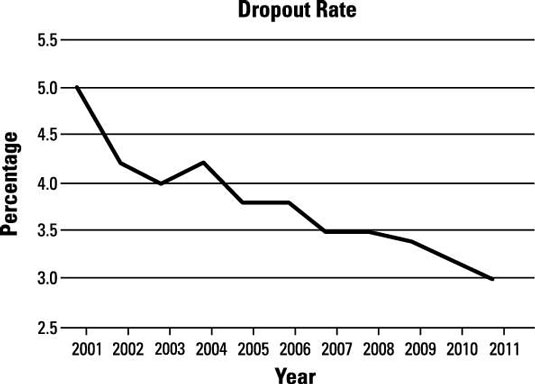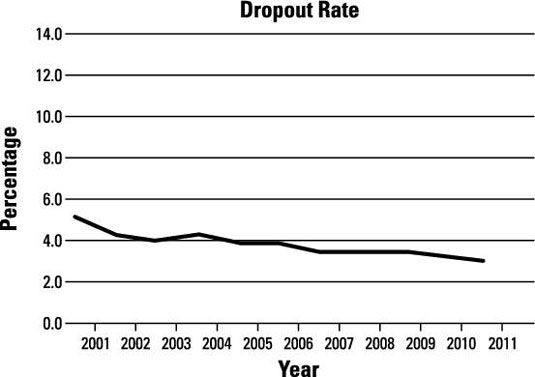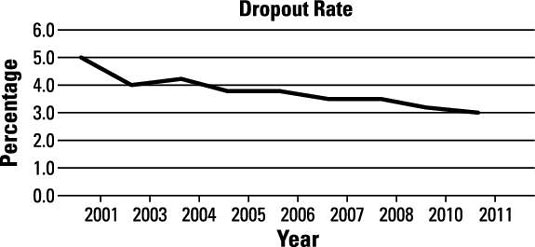##### Statistics: 1,001 Practice Problems For DummiesTime plots are used for highlighting data collected over time. The data in the following time chart shows the annual high-school dropout rate for a school system for the years 2001 to 2011.Credit: Illustration by Ryan Sneed

## Sample questions

1. What is the general pattern in the dropout rate from 2001 to 2011?

Although not every year shows a decrease from the previous year, the clear overall pattern of the dropout rate is one of a steady decrease.

2. What was the approximate dropout rate in 2005?

Find where the value for 2005 on the x-axis intersects with the value for the dropout rate on the y-axis; it's at 3.8%.

3. What was the approximate change in the dropout rate from 2001 to 2011?

Answer: drop of 2 percentage points

The dropout rate in 2001 was about 5%, and in 2011, it was about 3%. So the dropout rate from 2001 to 2011 is 3% – 5% = –2% which means there was a drop of 2 percentage points.

4. What was the approximate change in the dropout rate from 2003 to 2004?

Answer: rise of 0.2 percentage points.

The dropout rate in 2003 was about 4.0%, and in 2004, it was 4.2%. So the dropout rate from 2003 to 2004 changed by 4.2% – 4.0% = 0.2% which means there was an increase (rise) of 0.2 percentage points.

5. This time chart displays the same data, but why is it misleading?Credit: Illustration by Ryan Sneed

Answer: The y-axis scale has been stretched (the range of y-values goes well outside the data).

The change in dropout rates is made to look less significant in this chart because the y-axis scale runs from 0% to 14%, while the data runs from 3% to 5%.

6. Why do the numbers on this plot represent dropout rates instead of the number of dropouts?

Answer: because you need to take into account the number of students enrolled each year, not just the number who dropped out

Suppose that one year, out of 1,000 students in the system, 10 of them dropped out, so 10/1,000 = 0.01, or 1% of the students dropped out.

However, if the number of students in the system was only 500, but the number of dropouts was the same, then 10/500 = 0.02, or 2% of the students dropped out. Dividing by the total number of students calculates a dropout rate, which allows for a fair comparison.

7. This time chart displays the same data, but why is it misleading?Credit: Illustration by Ryan Sneed

Answer: Some points have been omitted from the scale of the x-axis.

Data for the years 2002 and 2009 have been omitted, but there's no indication of this omission on the chart (that is, no broken line calls attention to the missing years).

If you need more practice on this and other topics from your statistics course, visit 1,001 Statistics Practice Problems For Dummies to purchase online access to 1,001 statistics practice problems! We can help you track your performance, see where you need to study, and create customized problem sets to master your stats skills.